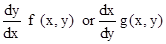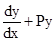# Differential Equations Class 12 Notes Mathematics

Differential Equations class 12 Notes Mathematics in PDF are available for free download in myCBSEguide mobie app. The best app for CBSE students now provides Differential Equations class 12 Notes latest chapter wise notes for quick preparation of CBSE board exams and school based annual examinations. Class 12 Mathematics notes on chapter 9 Differential Equations are also available for download in CBSE Guide website.

## Differential Equations Class 12 Notes Mathematics

Download CBSE class 12th revision notes for chapter 9 Differential Equations in PDF format for free. Download revision notes for Differential Equations class 12 Notes and score high in exams. These are the Differential Equations class 12 Notes prepared by team of expert teachers. The revision notes help you revise the whole chapter 9 in minutes. Revision notes in exam days is one of the best tips recommended by teachers during exam days.

## CBSE Class 12 Mathematics Revision Notes Chapter 9 Differential Equations

• Differential Equation: An equation involving derivatives of the dependent variable with respect to independent variable (variables) is known as a differential equation.
• Linear and non-linear differential equation: A differential equation is said to be linear if unknown function (dependent variable) as its derivative which occurs in the equation, occur only in the first degree and are not multiplied together. Otherwise the differential equaiton is said to be non-linear.
• Order: Order of a differential equation is the order of the highest order derivative occurring in the differential equation.
• Degree: Degree of a differential equation is defined if it is a polynomial equation in its derivatives.
• Degree (when defined) of a differential equation is the highest power (positive integer only) of the highest order derivative in it.
• Solution: A function which satisfies the given differential equation is called its solution.
• General Solution: The solution which contains as many arbitrary constants as the order of the differential equation is called a general solution.
• Particular Solution:  The solution free from arbitrary constants is called particular solution.
• To form a differential equation from a given function we differentiate the function successively as many times as the number of arbitrary constants in the given function and then eliminate the arbitrary constants.
• Variable Separable method: Variable separable method is used to solve such an equation in which variables can be separated completely i.e. terms containing y should remain with dy and terms containing x should remain with dx.
• A differential equation which can be expressed in the formwhere, f(x , y) and g (x , y) are homogenous functions of degree zero is called a homogeneous differential equation.
• A differential equation of the form= Q, where P and Q are constants or functions of x only is called a first order linear differential equation.

## CBSE Class-12 Revision Notes and Key Points

Differential Equations class 12 Notes. CBSE quick revision note for class-12 Chemistry Physics Math’s, Biology and other subject are very helpful to revise the whole syllabus during exam days. The revision notes covers all important formulas and concepts given in the chapter. Even if you wish to have an overview of a chapter, quick revision notes are here to do if for you. These notes will certainly save your time during stressful exam days.

To donload Differential Equations class 12 Notes, sample paper for class 12 Physics, Chemistry, Biology, History, Political Science, Economics, Geography, Computer Science, Home Science, Accountancy, Business Studies and Home Science; do check myCBSEguide app or website. myCBSEguide provides sample papers with solution, test papers for chapter-wise practice, NCERT Differential Equations, NCERT Exemplar Differential Equations, quick revision notes for ready reference, CBSE guess papers and CBSE important question papers. Sample Paper all are made available through the best app for CBSE students and myCBSEguide website.Test Generator

Create question paper PDF and online tests with your own name & logo in minutes.myCBSEguide

Question Bank, Mock Tests, Exam Papers, NCERT Solutions, Sample Papers, Notes